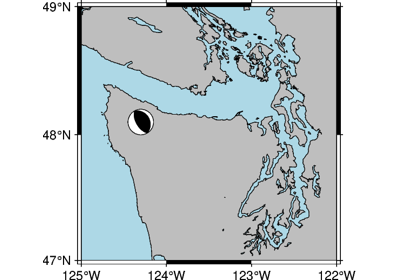# pygmt.Figure.meca¶

Figure.meca(spec, scale, longitude=None, latitude=None, depth=None, convention=None, component='full', plot_longitude=None, plot_latitude=None, *, region=None, projection=None, offset=None, frame=None, no_clip=None, verbose=None, xshift=None, yshift=None, panel=None, perspective=None, transparency=None, **kwargs)

Plot focal mechanisms.

Full option list at https://docs.generic-mapping-tools.org/latest/supplements/seis/meca.html

Note

Currently, labeling of beachballs with text strings is only supported via providing a file to spec as input.

Aliases:

• A = offset

• B = frame

• J = projection

• N = no_clip

• R = region

• V = verbose

• X = xshift

• Y = yshift

• c = panel

• p = perspective

• t = transparency

Parameters
• spec (dict, 1D array, 2D array, pd.DataFrame, or str) –

Either a filename containing focal mechanism parameters as columns, a 1- or 2-D array with the same, or a dictionary. If a filename or array, convention is required so we know how to interpret the columns/entries. If a dictionary, the following combinations of keys are supported; these determine the convention. Dictionary may contain values for a single focal mechanism or lists of values for many focal mechanisms. A Pandas DataFrame may optionally contain columns latitude, longitude, depth, plot_longitude, and/or plot_latitude instead of passing them to the meca method.

• `"aki"`strike, dip, rake, magnitude

• `"gcmt"`strike1, dip1, rake1, strike2, dip2, rake2, mantissa, exponent

• `"mt"`mrr, mtt, mff, mrt, mrf, mtf, exponent

• `"partial"`strike1, dip1, strike2, fault_type, magnitude

• `"principal_axis"`t_exponent, t_azimuth, t_plunge, n_exponent, n_azimuth, n_plunge, p_exponent, p_azimuth, p_plunge, exponent

• scale (str) – Adjusts the scaling of the radius of the beachball, which is proportional to the magnitude. Scale defines the size for magnitude = 5 (i.e. scalar seismic moment M0 = 4.0E23 dynes-cm)

• longitude (int, float, list, or 1d numpy array) – Longitude(s) of event location. Ignored if spec is not a dictionary. List must be the length of the number of events. Ignored if spec is a DataFrame and contains a ‘longitude’ column.

• latitude (int, float, list, or 1d numpy array) – Latitude(s) of event location. Ignored if spec is not a dictionary. List must be the length of the number of events. Ignored if spec is a DataFrame and contains a ‘latitude’ column.

• depth (int, float, list, or 1d numpy array) – Depth(s) of event location in kilometers. Ignored if spec is not a dictionary. List must be the length of the number of events. Ignored if spec is a DataFrame and contains a ‘depth’ column.

• convention (str) – `"aki"` (Aki & Richards), `"gcmt"` (global CMT), `"mt"` (seismic moment tensor), `"partial"` (partial focal mechanism), or `"principal_axis"` (principal axis). Ignored if spec is a dictionary or dataframe.

• component (str) – The component of the seismic moment tensor to plot. `"full"` (the full seismic moment tensor), `"dc"` (the closest double couple with zero trace and zero determinant), `"deviatoric"` (zero trace)

• plot_longitude (int, float, list, or 1d numpy array) – Longitude(s) at which to place beachball, only used if spec is a dictionary. List must be the length of the number of events. Ignored if spec is a DataFrame and contains a ‘plot_longitude’ column.

• plot_latitude (int, float, list, or 1d numpy array) – Latitude(s) at which to place beachball, only used if spec is a dictionary. List must be the length of the number of events. Ignored if spec is a DataFrame and contains a ‘plot_latitude’ column.

• offset (bool or str) – Offsets beachballs to the longitude, latitude specified in the last two columns of the input file or array, or by plot_longitude and plot_latitude if provided. A small circle is plotted at the initial location and a line connects the beachball to the circle. Specify pen and optionally append `+ssize` to change the line style and/or size of the circle.

• no_clip (bool) – Does NOT skip symbols that fall outside frame boundary specified by region [Default is False, i.e. plot symbols inside map frame only].

• projection (str) – Required if this is the first plot command. projcode[projparams/]width. Select map projection.

• region (str or list) – Required if this is the first plot command. xmin/xmax/ymin/ymax[+r][+uunit]. Specify the region of interest.

• frame (bool or str or list) – Set map boundary frame and axes attributes.

• verbose (bool or str) –

Select verbosity level [Default is w], which modulates the messages written to stderr. Choose among 7 levels of verbosity:

• q - Quiet, not even fatal error messages are produced

• e - Error messages only

• w - Warnings [Default]

• t - Timings (report runtimes for time-intensive algorithms);

• i - Informational messages (same as `verbose=True`)

• c - Compatibility warnings

• d - Debugging messages

• xshift (str) – [a|c|f|r][xshift]. Shift plot origin in x-direction.

• yshift (str) – [a|c|f|r][yshift]. Shift plot origin in y-direction. Full documentation is at https://docs.generic-mapping-tools.org/latest/gmt.html#xy-full.

• panel (bool or int or list) – [row,col|index]. Select a specific subplot panel. Only allowed when in subplot mode. Use `panel=True` to advance to the next panel in the selected order. Instead of row,col you may also give a scalar value index which depends on the order you set via `autolabel` when the subplot was defined. Note: row, col, and index all start at 0.

• perspective (list or str) – [x|y|z]azim[/elev[/zlevel]][+wlon0/lat0[/z0]][+vx0/y0]. Select perspective view and set the azimuth and elevation angle of the viewpoint. Default is [180, 90]. Full documentation is at https://docs.generic-mapping-tools.org/latest/gmt.html#perspective-full.

• transparency (int or float) – Set transparency level, in [0-100] percent range. Default is 0, i.e., opaque. Only visible when PDF or raster format output is selected. Only the PNG format selection adds a transparency layer in the image (for further processing).

## Examples using `pygmt.Figure.meca`¶